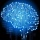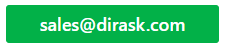Home
IT Knowledge
IT Career
Inspiration
Languages
EN

# C# / .NET - swap method

11 points
Created by:Root-ssh
68940

Swap operation in C#/.NET can be made in few ways.

## 1. Custom Swap method with reference arguments example

``````public static class SwapUtils
{
public static void Swap<T>(ref T a, ref T b)
{
T tmp = a;

a = b;
b = tmp;
}
}``````

Example:

``````int a1 = 3, b1 = 10;
int a2 = 4, b2 = 1;
int a3 = 5, b3 = 2;

SwapUtils.Swap(ref a1, ref b1);
SwapUtils.Swap(ref a2, ref b2);
SwapUtils.Swap(ref a3, ref b3);

Console.WriteLine("a1=" + a1 + ", b1=" + b1);
Console.WriteLine("a2=" + a2 + ", b2=" + b2);
Console.WriteLine("a3=" + a3 + ", b3=" + b3);``````

Output:

``````a1=10, b1=3
a2=1, b2=4
a3=2, b3=5``````

## 2. XOR swap algorithm example

``````int x = 3, y = 6;

x = x ^ y;
y = y ^ x;
x = x ^ y;

Console.WriteLine("x=" + x + ", y=" + y);``````
Note: this algorithm is useful with programming devices with very small amount of internal operating memory.

Output:

``x=6, y=3``

## 3. References